Next: Soundness of KT5-models Up: Kripke-type Semantics Previous: Kripke-type Semantics

## Definition of Kripke-type models

Let W be any non-empty set (of possible worlds). A model M on W is a triple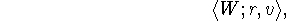where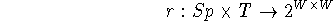and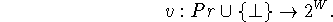Given any model M, we define a relation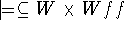as follows: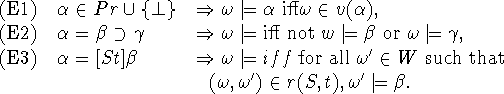We will write ``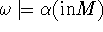'' if we wish to make M explicit. A formula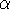is said to be valid in M, denoted by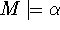, if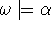for all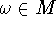. (By, we mean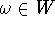.) Furthermore, we will employ the following notation: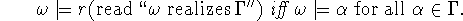A model M is a KT5-model if

(M1)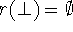(M2)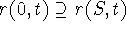for any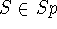and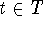,

(M3)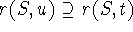for anyand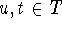such that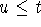,

(M4) r(S,t) is an equivalence relation for anyand.

A set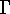of well formed formulas is said to be realizable if there exists a KT-5 model M andsuch that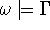.

Yasuko Kitajima
Fri Jun 20 13:39:43 PDT 1997